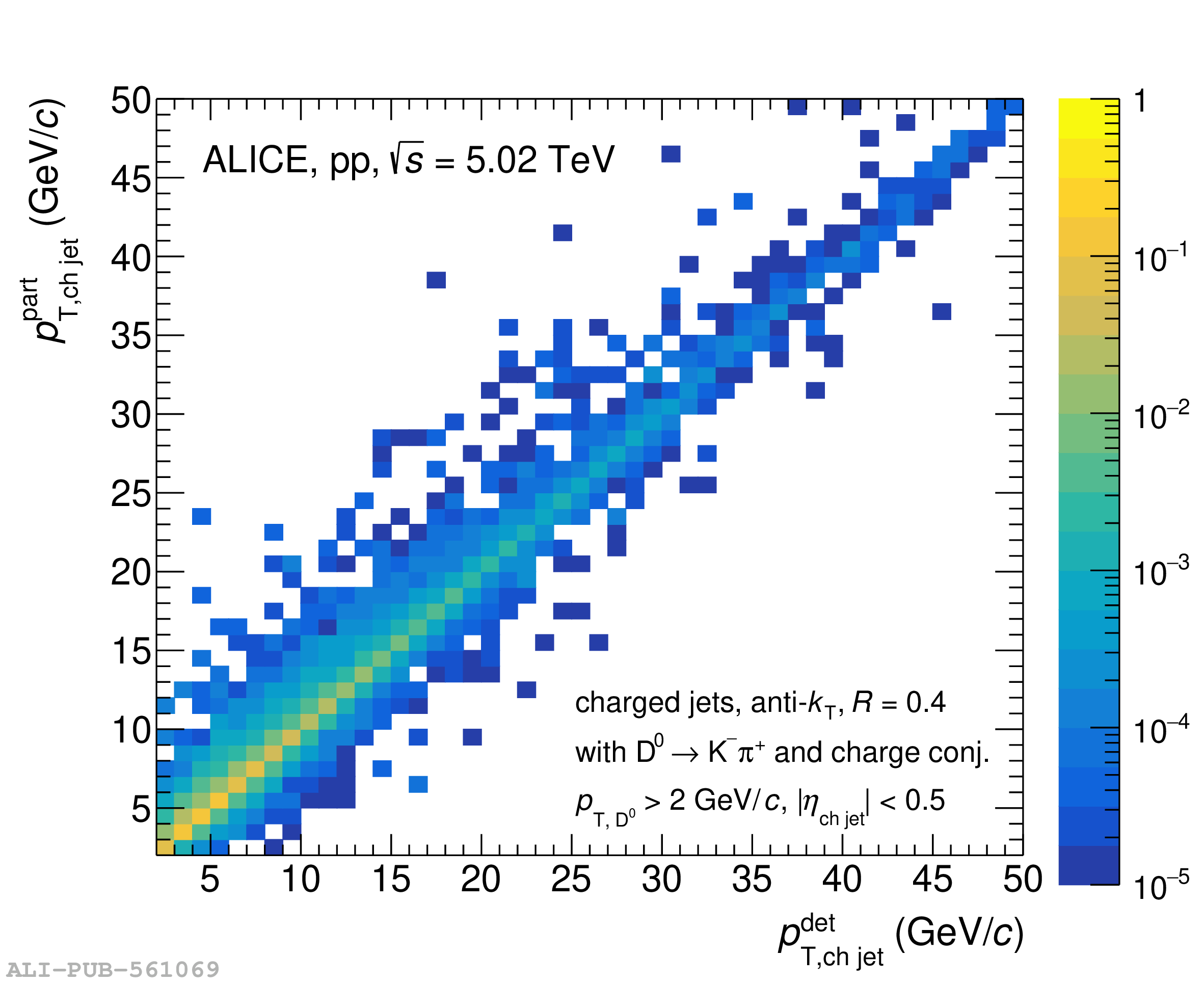# Figure 5

 Left: detector response matrix of matched jets used for unfolding the $p_{\mathrm{T,ch\,jet}}$ distribution with $R=0.4$ in pp collisions at $\sqrt{s}$ = 5.02 TeV in the range $2< p_{\mathrm{T,ch\,jet}} <50$ GeV/$c$. Right: $p_{\mathrm{T,ch\,jet}}$ resolution, $\Delta_{p}$, for $5< p_{\mathrm{T,ch\,jet}}^{\mathrm{part}} <6$ GeV/$c$, $10< p_{\mathrm{T,ch\,jet}}^{\mathrm{part}} <12$ GeV/$c$, and $20< p_{\mathrm{T,ch\,jet}}^{\mathrm{part}} <30$ GeV/$c$.